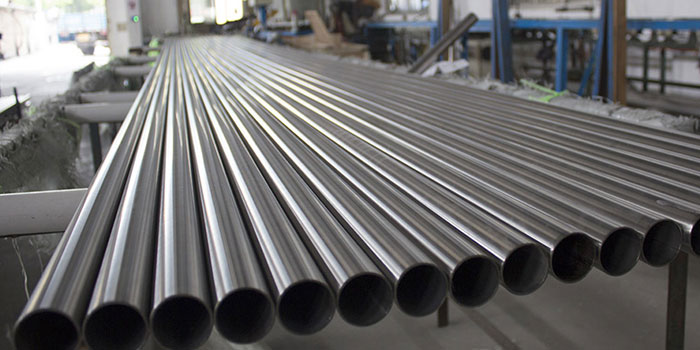11
Jul

### Understanding Pipe Dimensions

When you going to purchase pipes for your applications, the dealer or supplier ask which type of or what NPS & SCH of pipe dimensions? You know only Outside and Inside diameter. Then there is silence. You don’t know any of this, then supplier handover standard pipe charts to you, still you don’t know how to read, so we thought we would shed some light on the pipe chart you are viewing.

In Pipe chart, the first column is the “Size” which is Nominal Pipe Size (NPS). As per Wikipedia, Nominal Pipe Size (NPS) is the number that defines the size of the pipe. For example, when you say 10” (inch) pipe, means the 10” nominal pipe size of that pipe. The pipe dimensions have two non-dimensional numbers like Nominal Pipe Size (NPS) and Schedule (SCH).

Now, confused about Schedule (SCH), it is the way to indicate pipe wall thickness. It mainly depends on the ratio of service pressure in psi to allowable stress in psi. There is more confusion between these two numbers and actual pipe dimensions. If you asked for 2 inches 40 SCH pipe the actual diameter of the pipe is 2.375 inch and not 2 inches.

To explain how it works, you should keep some important points in mind i.e.

• NPS is not that related to inside diameter in inches but only for NPS 1/8” to NPS 12”
• For higher range, means more than 14”, NPS is equal to the outside diameter (OD) in inches.

Given, NPS, the outside diameter (OD) is constant and increasing wall thickness with an increase in Schedule (SCH). Given SCH, the outside diameter (OD) increases with increasing NPS however wall thickness may remain the same or increases. In this way this chart in documented.

There are various pipe dimensions schedule are used today include 40, 80 and 160. SCH 40 is dominating in an industry that doesn’t mean it is standard schedule dimensions. For NPS 12” and larger SCH 40 is variable wall thickness.

There are different standards for pipe schedule are available in the market, all are not identical. So, you will feel confused, so don’t worry keep one thing in mind that Schedule is used to indicated wall thickness. You get wall thickness from SCH and Outside Diameter from NPS then, you can easily calculate inside diameter of pipe using the following formula.

Pipe Inside Diameter = Pipe Outside Diameter – (2 x Pipe wall thickness)

For example, we select NPS 14” Schedule 40 Pipe, now we have to find the Inside diameter of the pipe.

Here you get the Outside Diameter of Pipe from NPS i.e. 14 = 14 inch & wall thickness from Schedule (SCH) i.e. 40 = 0.438 inch

To calculate inside diameter, we use the following formula

Pipe Inside Diameter = Pipe Outside Diameter – (2 x Pipe wall thickness)

After calculations, Pipe inside diameter = 13.124 inch